# Range Of A Function (6 Common Questions Answered)

When we talk about functions in math, we want to know what set of output values are valid and possible (known as the range or image of the function).  Knowing the range of a function also helps us to figure out the codomain (a set that contains the range).

So, what do you need to know about the range of a function?  The range of a function f(x) is the set of outputs (there is an input “x” that yields the output “y” so that f(x) = y). The range of a function varies, depending on whether the function is complex-valued, real-valued, etc. Values of y in a range can be repeated (2 or more inputs have the same output).

Of course, range can vary quite a bit, from the entire set of real numbers to a single element (such as zero).  We need to examine the function itself and do some thinking to figure out its range.

In this article, we’ll talk about the range of a function and what it is.  We’ll also answer some common questions about range and go through some examples to make the concept clear.

Let’s get started.

## What Is The Range Of A Function?

For a function f(x), its range is the entire set of possible output values.  That is, for every value of y in the range, there is an x in the domain so that f(x) = y.

The notation we often use is f:A->B, where:

• A is the domain of f(x) (input values)
• B is the range of f(x) (output values)

For example, if we have the function f(x) = x2 and a domain of A = {0, 1, 2}, then the range is B = {0, 1, 4} (since f(0) = 02 = 0, f(1) = 12 = 1, and f(2) = 22 = 4).

If we take f(x) = x2 with a domain of real numbers, then the range is the set of nonnegative real numbers (since a real number squared is never negative, but can be zero or positive).

The range of a function can vary, depending on whether the function is complex-valued, real-valued, or otherwise.

For example, if the function f(x) = √x is real-valued, then it has a range of nonnegative real numbers (since the domain is x >= 0).  The reason is that for any negative x, √x is imaginary.

On the other hand, if the function f(x) = √x is complex-valued, then it has a range of RuI (real numbers and imaginary numbers).  This is because imaginary outputs are allowed for a complex-valued function, and for any imaginary number bi, the input x = -b2 will give an output of bi.

Note: if both the domain and range are subsets of the real numbers, then f(x) can be graphed on a 2-dimensional coordinate plane.

### Can Domain & Range Be The Same?

Domain and range can be the same in certain situations.

One situation when domain and range are the same is when a function is its own inverse (called an involution).  That is:

• f(x) = f-1(x), or
• f(f(x)) = x

Some such involution functions include:

• f(x) = x  (Also called the identity function.  Both the domain and range are the entire set of real numbers).
• f(x) = a/x for any real number a  (Also called the reciprocal function for a = 1.  Both the domain and range are the set of nonzero real numbers, or R – {0}).
• f(x) = -x + b for any real number b  (These are linear functions with a slope of -1.  Both the domain and range are the entire set of real numbers.  Note that this includes f(x) = -x, where b = 0).

There are many other functions (besides involutions) where the domain and range are the same.

For example, the real-valued function f(x) = x3 has a domain and range of all real numbers.  (The same is true for any real-valued function g(x) = xM where M is an odd integer.

The inverses of these functions (like f-1(x) = x1/3 or g-1(x) = x1/M) also have domain and range of all real numbers.

Another example is the real-valued function f(x) = |√x|.  Because of the radical, we can only have nonnegative numbers in the domain, and because of the absolute value symbol, we can only have nonnegative numbers in the range.

So, the domain and range are the same: the set of nonnegative real numbers.

### Can Range Repeat In A Function?

Range can repeat in a function that is not one-to-one.  However, range does not repeat in a one-to-one function.

Remember, a one-to-one function is a specific type of function: one where two different inputs x1 and x2 map to two different outputs y1 = f(x1) and y2 = f(x2).

We can tell that the graph of a function is one-to-one with the horizontal line test.  This test states that if there is any horizontal line that intersects the graph of the function more than once, then the function is not one-to-one; otherwise, it is one-to-one.

So, for a function that is not one-to-one, the range can repeat.

#### Example 1: A Function That Is Not One-To-One (Repeated Values In Range)

Consider the function f(x) = x2.  This function is not one-to-one, since it fails the horizontal line test (we can see this in the graph below for the line y = 1).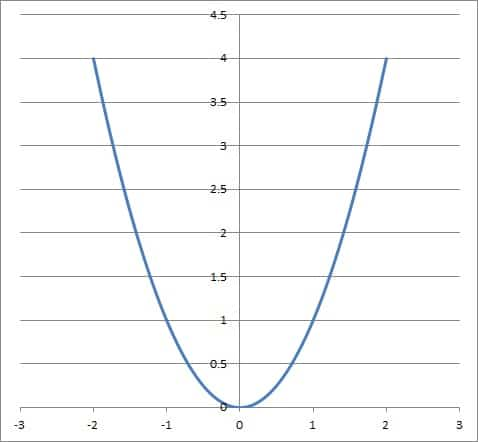The graph of the function f(x) = x2. This function is not one-to-one, since the horizontal line y = 1 intersects the graph at x = 1 and x = -1.

We can also see this with values x = 1 and x = -1.  Both have an output of 1, since f(1) = 12 = 1 and f(-1) = (-1)2 = 1.

#### Example 2: A Function That Is One-To-One (No Repeated Values In Range)

Consider the function f(x) = x.  This function is one-to-one, since there is no horizontal line that intersects its graph twice (we can see this in the graph below).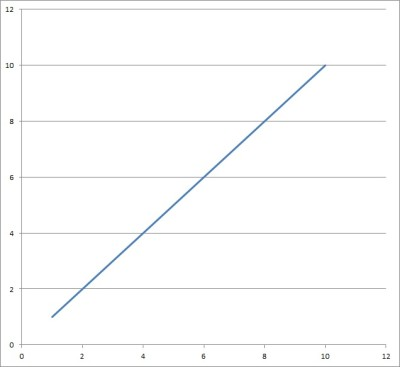The graph of the function f(x) = x. This function is one-to-one, since there is no horizontal line that intersects the graph more than once.

We can also see this by choosing any value of y (output).  When we solve y = f(x), we get y = x, and so there is only one value of x (input) that leads to an output of y.

### Can Range Be All Real Numbers?

The range of a function can be the set of all real numbers.  There are several examples where this is true:

• f(x) = xN, where N is a positive odd integer.  (Whatever real value of x we choose, we just multiply it by itself N times to get a valid output f(x)).
• f(x) = ax + b, where a and b are real numbers and a is not zero (linear functions).
• f(x) = ax3 + bx2 + cx + d, where a, b, c, and d are real numbers and a is not zero (cubic functions).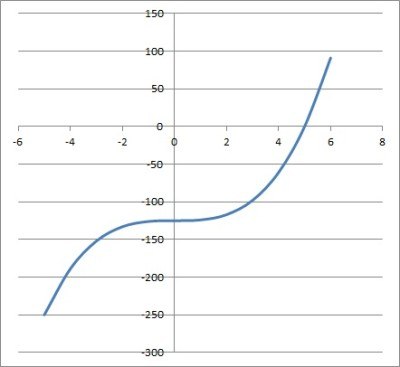The graph of the cubic function f(x) = x3 – 125. The range of this function is all real numbers.

This list is not exhaustive; there are many more examples besides the ones above.

### Can Range Be Zero?

The range of a function can be just the number zero.  For example, if we have the real-valued function f(x) = √(-x2), then the domain is the number zero {0}, and the range is the number zero {0}.  Every other real number input would lead to an imaginary output.

The range of a function can include zero in some cases.  There are also cases where zero is excluded from the range.

#### Example 1: A Function With A Range Of Zero

Consider the real-valued function f(x) = √(-x4).  The range of this function is the set {0}, or just the number zero.

Every other real number leads to an imaginary output, since:

• x4 is positive for any real x that is not zero
• -x4 is negative for any real x that is not zero
• f(x) = √(-x4) is imaginary for any real x that is not zero

#### Example 2: A Function Whose Range Excludes Zero

Consider the real-valued function f(x) = 1/x.  The range of this function is the set R – {0}, or the set of nonzero real numbers.

For y = 0, we have to solve 0 = 1/x, which has no real solution.  So, y = 0 is not in the range of the function y = 1/x.

For any other real number y, we solve y = 1/x to get x = 1/y, which is defined and real for y not zero.

You can see the graph of this function below.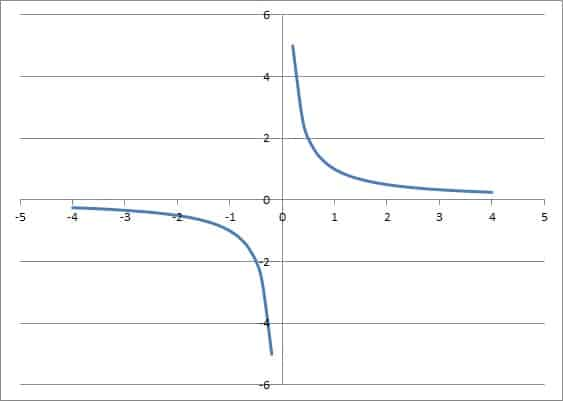The graph of the function f(x) = 1/x has a range of all real numbers except y = 0.

### Can Range Be Negative?

In some cases, the range of a function can be entirely made up of negative numbers.  For example, the real-valued function f(x) = -√(|x| + 1)  has the domain y <= -1, which is entirely made up of negative numbers.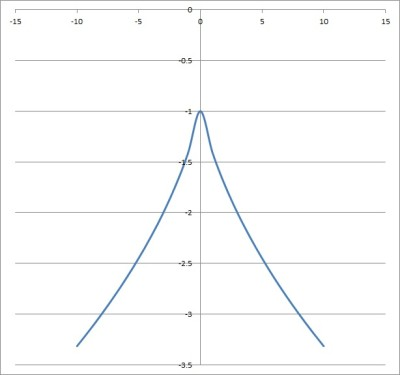The graph of the function f(x) = -√(|x| + 1) has a range of y <= -1.

Of course, a function’s range can also include some negative numbers, but also some positive numbers and/or zero.

The key thing to remember is that the square root of a negative number is imaginary (which is undefined for a real-valued function).

In fact, any even root (fourth root, sixth root, eight root, etc.) of a negative number leads to a complex number with a nonzero imaginary part.

To prove this, assume we are taking an Nth root, where N is an even integer.  Then N = 2M, where M is an integer.  Then:

• x1/N
• =x1/2M
• =(x1/2)1/M
• =(√x)1/M

So, taking an even root of x amounts to taking a square root of x.

#### Example 1: A Function With A Negative Range

Consider the real-valued function f(x) = -√(|x| + 4).  The range of this function is the set y <= -2, which includes only negative numbers.

We can see this as follows:

• |x| >= 0  [by definition of absolute value]
• |x| + 4 >= 0 + 4
• √(|x| + 4) >= √4
• -√(|x| + 4) <= -2  [flip inequality after multiplying by -1]
• f(x) <= -2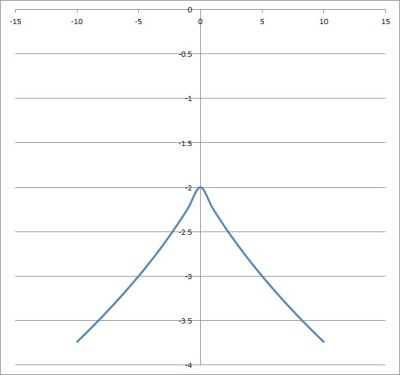The graph of the function f(x) = -√(|x| + 1) has a range of y <= -2.

#### Example 2: A Function Whose Range Excludes Negative Numbers

Consider the real-valued function f(x) = √(x-2).  The range of this function is the set y >= 0, which excludes negative numbers.

By convention, when we find the square root of a positive real number, we take the principal (positive) square root.  For example, both 2 and -2 are square roots of 4, but we take 2 (positive) as the principal square root of 4.

• x >= 2  [domain for the function]
• x – 2 >= 0
• √(x-2) >= 0
• f(x) >= 0

### How To Find The Range Of A Function

To find the range of a real-valued function, take the following steps:

You can also find the inverse of the function f(x) by replacing f(x) with y, solving for x, switching the variables x and y, and replacing y with f-1(x).  Then, the domain of f-1(x) is the range of f(x).  (However, not all functions are invertible, so this method may not always work).

Here are some examples of how to find the domain of a function.

#### Example 1: Finding the Range Of A Function

Consider the real-valued function f(x) = 1 / (x – 1)(x – 2).  The range of this function is the set of real numbers y > 0 and y <= -4.

We can see this by finding the domain first (solving for a zero denominator):

• (x – 1)(x – 2) = 0
• x – 1 = 0 OR x – 2 = 0
• x = 1 OR x = 2

So, the domain is the set of all real numbers excluding x = 1 and x = 2, or the set R – {1, 2}.

For a given real value of y (output), we can try to solve f(x) = y:

• f(x) = y
• 1 / (x – 1)(x – 2) = y
• 1 = (x – 1)(x – 2)y
• 1 = (x2 – 3x + 2)y
• 0 = (y)x2 + (– 3y)x + (2y – 1)

This is a quadratic equation of the form ax2 + bx + c = 0, with a = y, b = -3y, and c = 2y – 1.

Using the quadratic formula, we get y2 + 4y for the discriminant (the expression under the radical).  Since we are looking for real values of x, we want y2 + 4y >= 0, or y(y + 4) >= 0.

This is only true in two cases:

• y >= 0 and y + 4 >= 0, meaning y >= 0 and y >= -4, or y >= 0
• y <=  0 and y + 4 <= 0, meaning y <= 0 and y <= -4, or y <= -4.

Of course, we must exclude the value y = 0 from the range, since there is no solution for f(x) = 0:

• f(x) = 0
• 1 / (x – 1)(x – 2) = 0
• 1 = 0  [no solution]

We can verify the range of f(x) with the graph below.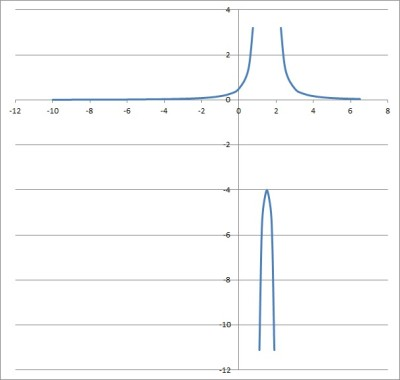The graph of the function f(x) = 1 / (x – 1)(x – 2) has a range of y > 0 and y <= -4.

#### Example 2: Finding the Range Of A Function

Consider the real-valued function f(x) = 4√(x – 3).  The range of this function is the set y >= 0.

We can see this by finding the domain first (solving for a negative radicand):

• x – 3 < 0
• x < 3 (these numbers are excluded from the domain, since they result in an even root of a negative number).

So, the domain is the set of real numbers x >= 3.

At x = 3, we get f(3) = 4√(3 – 3) = 0.

For any x >= 3, we get a positive real number for x – 3.  The principal fourth root of this number will be a positive real number, so y >= 0 for any real x >= 3.

We can verify the range of f(x) with the graph below.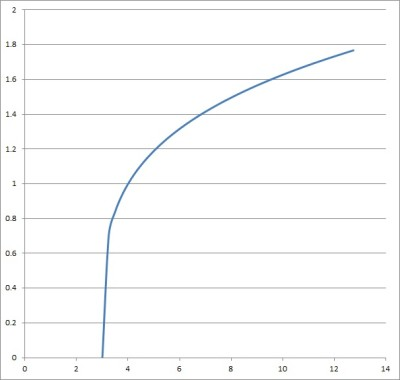The graph of the function f(x) = 4√(x – 3) has a range of y >= 0.

## Conclusion

Now you know about the range of a function and how to find it, along with the answers to some common questions.

You can learn about the differences between domain & range here.